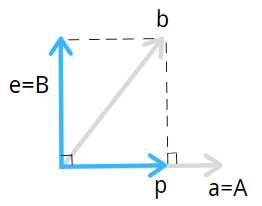# 线性代数17.正交矩阵和格拉姆-施密特

## 正交基

$$q_1、q_2、q_3...q_n$$ 表示标准正交基，标准表示长度是单位长度，任何 $$q$$ 都与其他 $$q$$ 正交，她具有性质：

$q_i^T.q_j= \begin{array}{cc} \{ & \begin{array}{cc} 1 & i\neq j \ 0 & i=j \\end{array} \\end{array}$

## 正交矩阵

$Q=\left( \begin{array}{cccc} q_1 & q_2 & ... & q_n \\end{array} \right)$

$Q^TQ=\left( \begin{array}{c} q_1 \ q_2 \ ... \ q_n \\end{array} \right).\left( \begin{array}{cccc} q_1 & q_2 & ... & q_n \\end{array} \right) =\left( \begin{array}{cccc} 1 & 0 & ... & 0 \ 0 & 1 & ... & 0 \ ... & ... & ... & ... \ 0 & 0 & 0 & 1 \\end{array} \right)=I$

### 举例1

$perm Q=\left( \begin{array}{ccc} 0 & 0 & 1 \ 1 & 0 & 0 \ 0 & 1 & 0 \\end{array} \right)$

$$Q^TQ$$

$\left( \begin{array}{ccc} 0 & 1 & 0 \ 0 & 0 & 1 \ 1 & 0 & 0 \\end{array} \right).\left( \begin{array}{ccc} 0 & 0 & 1 \ 1 & 0 & 0 \ 0 & 1 & 0 \\end{array} \right)=I$

### 举例2

$Q=\left( \begin{array}{cc} \cos \theta & -\sin \theta \ \sin \theta & \cos \theta \\end{array} \right)、Q=\frac{1}{\sqrt{2}}\left( \begin{array}{cc} 1 & 1 \ 1 & -1 \\end{array} \right)、Q=\frac{1}{2} \left( \begin{array}{cccc} 1 & 1 & 1 & 1 \ 1 & -1 & 1 & -1 \ 1 & 1 & 1 & 1 \ 1 & -1 & 1 & -1 \\end{array} \right)$

### 举例3

$$Q$$ 当然也可以是长方矩阵。

$Q=\frac{1}{3} \left( \begin{array}{ccc} 1 & -2 & 2 \ 2 & -1 & -2 \ 2 & 2 & 1 \\end{array} \right)$

## 意义

\begin {align} P&=Q(Q^TQ)^{-1}Q^T\&=QQ^T \end {align}

\begin {align} P&=QQ^T\&=I \end {align}

\begin {align} A^TA\hat x=A^T b \end {align}

\begin {align} Q^TQ\hat x&=Q^T b\\rightarrow I\hat x&=Q^T b \end {align}

$\hat x_i=q_i^T.b$

## Graham-Schmidt正交化法

### 两列线性无关向量\begin {align} A&=a\B&=e\&=b-p\&=b-xa\&=b-\frac{ A^TA}{ A^Tb}A \end {align}

$q_1=\frac{ A}{ ||A||}\q_2=\frac{ B}{ ||B||}\$

### 三列线性无关向量

$$A、B$$ 同两列线性无关向量一样，

\begin {align} A&=a\B&=e\&=b-p\&=b-xa\&=b-\frac{ A^Tb}{ A^TA}A \end {align}

$$B$$ 其实是向量 $$b$$ 减去其在 $$a$$ 方向上的投影。

$C =c-\frac{ A^Tc}{ A^TA}A-\frac{ B^Tc}{ B^TB}B$

$q_1=\frac{ A}{ ||A||}\q_2=\frac{ B}{ ||B||}\q_3=\frac{ C}{ ||C||}\$

### 举例

$a=\left( \begin{array}{c} 1 \ 1 \ 1 \\end{array} \right),b=\left( \begin{array}{c} 1 \ 0 \ 2 \\end{array} \right)$

$A=\left( \begin{array}{c} \frac{1}{\sqrt{3}} \ \frac{1}{\sqrt{3}} \ \frac{1}{\sqrt{3}} \\end{array} \right),B=\left( \begin{array}{c} 1 \ 0 \ 2 \\end{array} \right)-\frac{3}{3} \left( \begin{array}{c} 1 \ 1 \ 1 \\end{array} \right)=\left( \begin{array}{c} 0 \ -1 \ 1 \\end{array} \right)$

$Q=\left( \begin{array}{cc} \frac{1}{\sqrt{3}} & 0 \ \frac{1}{\sqrt{3}} & -1 \ \frac{1}{\sqrt{3}} & 1 \\end{array} \right)$

$D=\left( \begin{array}{cc} 1 & 1 \ 1 & 0 \ 1 & 2 \\end{array} \right)$

$$D$$$$Q$$ 的列空间其实是一样的，都是同一个平面，因为 $$B$$$$a、b$$ 的线性组合，$$A$$$$a$$ 的线性组合。我们的计算只是让矩阵的基标准正交而已。

### 表达式

$A=QR$

$\left( \begin{array}{cc} a_1 & a_2 \\end{array} \right)=\left( \begin{array}{cc} q_1 & q_2 \\end{array} \right).\left( \begin{array}{cc} a_1^T q_1 & a_2^T q_1\ a_1^T q_2& a_2^T q_2\\end{array} \right)=\left( \begin{array}{cc} q_1 & q_2 \\end{array} \right).\left( \begin{array}{cc} a_1^T q_1 & a_2^T q_1\ 0& a_2^T q_2\\end{array} \right)$

$$a、q$$ 都表示列向量。